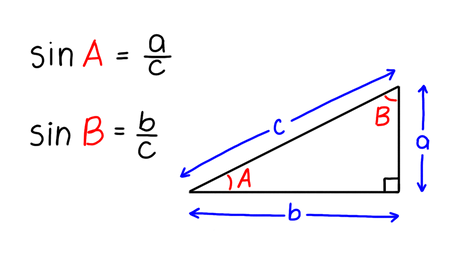VIEW IN FULL SCREEN

OCR GCSE Maths Sine1. Sine

Sine of an angle is a ratio between the side opposite to the angle and the hypotenuse of a triangle.1

Find sinB if b = 5 and c = 25.2

Find sinA if a = 7 cm and c = 10 cm.3

Find a if sinA = 0.5 and c = 8.4

Find a if sinA = 0.85 and c = 2 mm.5

Find b if sinB = 0.145 and c = 100 cm.End of page